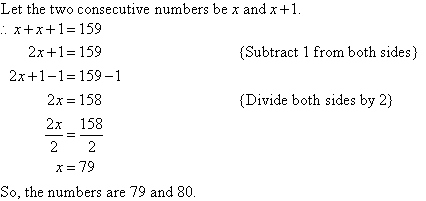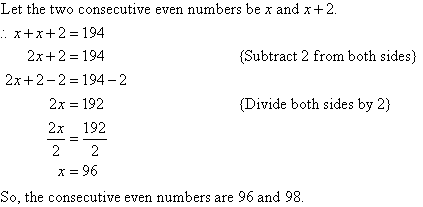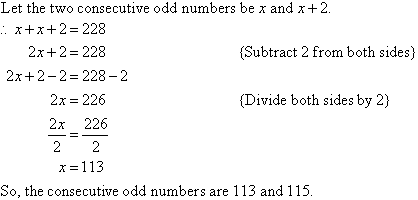Year 10 Interactive Maths - Second Edition

## Consecutive Numbers

If x is any number, then x and x + 1 are consecutive numbers.
E.g. 14 and 15 are consecutive numbers, as are 21 and 22.

#### Example 18

If the sum of two consecutive numbers is 159, find the numbers.

##### Solution:79 + 80 = 159

## Consecutive Even Numbers

If x is any even number, then x and x + 2 are consecutive even numbers.
E.g. 8 and 10 are consecutive even numbers, as are 24 and 26.

#### Example 19

If the sum of two consecutive even numbers is 194, find the numbers.

##### Solution:96 + 98 = 194

## Consecutive Odd Numbers

If x is any odd number, then x and x + 2 are consecutive odd numbers.
E.g.  7 and 9 are consecutive odd numbers, as are 31 and 33.

#### Example 20

If the sum of two consecutive odd numbers is 228, find the numbers.

##### Solution:###### Check:

113 + 115 = 228

Copyright © 2000-2019 mathsteacher.com Pty Ltd.  All rights reserved.
Australian Business Number 53 056 217 611

Copyright instructions for educational institutions

Please read the Terms and Conditions of Use of this Website and our Privacy and Other Policies.
If you experience difficulties when using this Website, tell us through the feedback form or by phoning the contact telephone number.